# Cluster Graph¶

class pgmpy.models.ClusterGraph.ClusterGraph(ebunch=None)[source]

Base class for representing Cluster Graph.

Cluster graph is an undirected graph which is associated with a subset of variables. The graph contains undirected edges that connects clusters whose scopes have a non-empty intersection.

Formally, a cluster graph is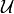for a set of factors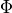over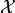is an undirected graph, each of whose nodesis associated with a subset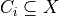. A cluster graph must be family-preserving - each factormust be associated with a cluster C, denoted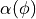, such that. Each edge between a pair of clusters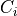and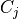is associated with a sepset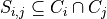.

Parameters:

data (input graph) – Data to initialize graph. If data=None (default) an empty graph is created. The data is an edge list

Examples

Create an empty ClusterGraph with no nodes and no edges

>>> from pgmpy.models import ClusterGraph
>>> G = ClusterGraph()


G can be grown by adding clique nodes.

Nodes:

Add a tuple (or list or set) of nodes as single clique node.

>>> G.add_node(('a', 'b', 'c'))
>>> G.add_nodes_from([('a', 'b'), ('a', 'b', 'c')])


Edges:

G can also be grown by adding edges.

>>> G.add_edge(('a', 'b', 'c'), ('a', 'b'))


or a list of edges

>>> G.add_edges_from([(('a', 'b', 'c'), ('a', 'b')),
...                   (('a', 'b', 'c'), ('a', 'c'))])


Add an edge between two clique nodes.

Parameters:
• u (nodes) – Nodes can be any list or set or tuple of nodes forming a clique.

• v (nodes) – Nodes can be any list or set or tuple of nodes forming a clique.

Examples

>>> from pgmpy.models import ClusterGraph
>>> G = ClusterGraph()
>>> G.add_nodes_from([('a', 'b', 'c'), ('a', 'b'), ('a', 'c')])
>>> G.add_edges_from([(('a', 'b', 'c'), ('a', 'b')),
...                   (('a', 'b', 'c'), ('a', 'c'))])


Associate a factor to the graph. See factors class for the order of potential values

Parameters:

*factor (pgmpy.factors.factors object) – A factor object on any subset of the variables of the model which is to be associated with the model.

Returns:

Return type:

None

Examples

>>> from pgmpy.models import ClusterGraph
>>> from pgmpy.factors.discrete import DiscreteFactor
>>> student = ClusterGraph()
>>> factor = DiscreteFactor(['Alice', 'Bob'], cardinality=[3, 2],
...                 values=np.random.rand(6))


Add a single node to the cluster graph.

Parameters:

node (node) – A node should be a collection of nodes forming a clique. It can be a list, set or tuple of nodes

Examples

>>> from pgmpy.models import ClusterGraph
>>> G = ClusterGraph()


Add multiple nodes to the cluster graph.

Parameters:

nodes (iterable container) – A container of nodes (list, dict, set, etc.).

Examples

>>> from pgmpy.models import ClusterGraph
>>> G = ClusterGraph()
>>> G.add_nodes_from([('a', 'b'), ('a', 'b', 'c')])

check_model()[source]

Check the model for various errors. This method checks for the following errors.

• Checks if factors are defined for all the cliques or not.

• Check for running intersection property is not done explicitly over here as it done in the add_edges method.

• Checks if cardinality information for all the variables is available or not. If not it raises an error.

• Check if cardinality of random variable remains same across all the factors.

Returns:

check – True if all the checks are passed

Return type:

boolean

copy()[source]

Returns a copy of ClusterGraph.

Returns:

ClusterGraph

Return type:

copy of ClusterGraph

Examples

>>> from pgmpy.factors.discrete import DiscreteFactor
>>> G = ClusterGraph()
>>> phi1 = DiscreteFactor(['a', 'b'], [2, 2], np.random.rand(4))
>>> phi2 = DiscreteFactor(['b', 'c'], [2, 2], np.random.rand(4))
>>> graph_copy = G.copy()
>>> graph_copy.factors
[<DiscreteFactor representing phi(a:2, b:2) at 0xb71b19cc>,
<DiscreteFactor representing phi(b:2, c:2) at 0xb4eaf3ac>]
>>> graph_copy.edges()
[(('a', 'b'), ('b', 'c'))]
>>> graph_copy.nodes()
[('a', 'b'), ('b', 'c')]

get_cardinality(node=None)[source]

Returns the cardinality of the node

Parameters:

node (any hashable python object (optional)) – The node whose cardinality we want. If node is not specified returns a dictionary with the given variable as keys and their respective cardinality as values.

Returns:

int or dict – If node is not specified returns a dictionary with the given variable as keys and their respective cardinality as values.

Return type:

If node is specified returns the cardinality of the node.

Examples

>>> from pgmpy.models import ClusterGraph
>>> from pgmpy.factors.discrete import DiscreteFactor
>>> student = ClusterGraph()
>>> factor = DiscreteFactor(['Alice', 'Bob'], cardinality=[2, 2],
...                 values=np.random.rand(4))
>>> student.get_cardinality()
defaultdict(<class 'int'>, {'Alice': 2, 'Bob': 2})

>>> student.get_cardinality(node='Alice')
2

get_factors(node=None)[source]

Return the factors that have been added till now to the graph.

If node is not None, it would return the factor corresponding to the given node.

Examples

>>> from pgmpy.models import ClusterGraph
>>> from pgmpy.factors.discrete import DiscreteFactor
>>> G = ClusterGraph()
>>> G.add_nodes_from([('a', 'b', 'c'), ('a', 'b'), ('a', 'c')])
>>> G.add_edges_from([(('a', 'b', 'c'), ('a', 'b')),
...                   (('a', 'b', 'c'), ('a', 'c'))])
>>> phi1 = DiscreteFactor(['a', 'b', 'c'], [2, 2, 2], np.random.rand(8))
>>> phi2 = DiscreteFactor(['a', 'b'], [2, 2], np.random.rand(4))
>>> phi3 = DiscreteFactor(['a', 'c'], [2, 2], np.random.rand(4))
>>> G.get_factors()
>>> G.get_factors(node=('a', 'b', 'c'))

get_partition_function()[source]

Returns the partition function for a given undirected graph.

A partition function is defined as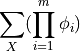where m is the number of factors present in the graph and X are all the random variables present.

Examples

>>> from pgmpy.models import ClusterGraph
>>> from pgmpy.factors.discrete import DiscreteFactor
>>> G = ClusterGraph()
>>> G.add_nodes_from([('a', 'b', 'c'), ('a', 'b'), ('a', 'c')])
>>> G.add_edges_from([(('a', 'b', 'c'), ('a', 'b')),
...                   (('a', 'b', 'c'), ('a', 'c'))])
>>> phi1 = DiscreteFactor(['a', 'b', 'c'], [2, 2, 2], np.random.rand(8))
>>> phi2 = DiscreteFactor(['a', 'b'], [2, 2], np.random.rand(4))
>>> phi3 = DiscreteFactor(['a', 'c'], [2, 2], np.random.rand(4))
>>> G.get_partition_function()

remove_factors(*factors)[source]

Removes the given factors from the added factors.

Examples

>>> from pgmpy.models import ClusterGraph
>>> from pgmpy.factors.discrete import DiscreteFactor
>>> student = ClusterGraph()
>>> factor = DiscreteFactor(['Alice', 'Bob'], cardinality=[2, 2],
...                         values=np.random.rand(4))